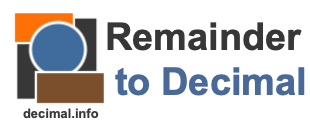14 remainder 3 as a decimalHere we will show you how to convert 14 remainder 3 into a decimal number. This is helpful if your answer to a long division problem was 14 remainder 3 (14R3), and you want to know what your answer is as a decimal.

14 remainder 3 as a decimal means that we want to convert 14R3 into a decimal number with an integer part, a decimal point, and a fractional part.

The integer part of 14R3 is the number that appears before the R. Therefore, the integer part is 14. To find the fractional part, we divide the remainder (the number that appears after the R, which is 3) by the divisor in the original division problem.

This means that we need the divisor in your division problem in order to determine what 14 remainder 3 is as a decimal number. Note that the divisor is always greater than the remainder. Please enter the divisor below and press "Decimal" to convert 14R3 to a decimal.

Remainder to Decimal Calculator
Go here to convert another remainder to a decimal number.

14 remainder 4 as a decimal
Here is a similar problem that we have explained and solved for you.